# Moment of Inertia: Cylinder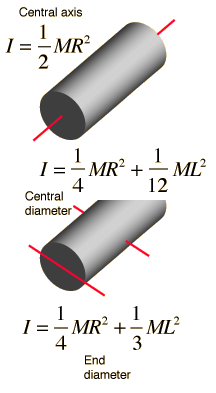A solid cylinder of
mass m= kg
will have a moment of inertia about its central axis:
 Icentral axis = kg m2

For a cylinder of
length L = m, the moments of inertia of a cylinder about other axes are shown.
 Icentral diameter = kg m2 Iend diameter = kg m2

The moments of inertia for the limiting geometries with this mass are:
 Ithin disk diameter = kg m2 Ithin rod end = kg m2

### Hollow cylinder case

Index

Moment of inertia concepts

 HyperPhysics***** Mechanics R Nave
Go Back

# Moment of Inertia: Cylinder

The expression for the moment of inertia of a solid cylinder can be built up from the moment of inertia of thin cylindrical shells.Using the general definition for moment of inertia: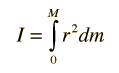The mass element can be expressed in terms of an infinitesmal radial thickness dr by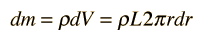Substituting gives a polynomial form integral:### Show form of integral

Index

Moment of inertia concepts

 HyperPhysics***** Mechanics R Nave
Go Back

# Moment of Inertia: Hollow Cylinder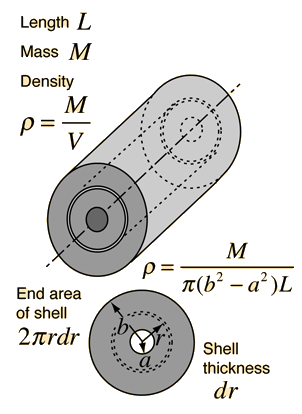The expression for the moment of inertia of a hollow cylinder or hoop of finite thickness is obtained by the same process as that for a solid cylinder. The process involves adding up the moments of infinitesmally thin cylindrical shells. The only difference from the solid cylinder is that the integration takes place from the inner radius a to the outer radius b: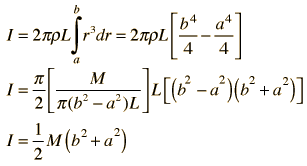### Show development of thin shell integral

Index

Moment of inertia concepts

 HyperPhysics***** Mechanics R Nave
Go Back

# Moment of Inertia: Cylinder About Perpendicular Axis

The development of the expression for the moment of inertia of a cylinder about a diameter at its end (the x-axis in the diagram) makes use of both the parallel axis theorem and the perpendicular axis theorem. The approach involves finding an expression for a thin disk at distance z from the axis and summing over all such disks.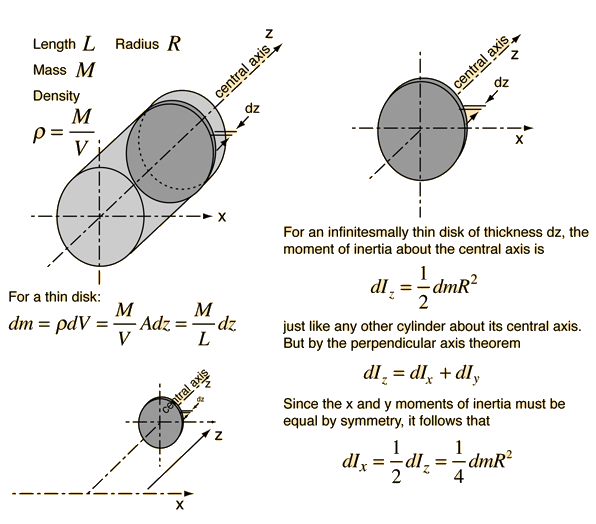Obtaining the moment of inertia of the full cylinder about a diameter at its end involves summing over an infinite number of thin disks at different distances from that axis. This involves an integral from z=0 to z=L. For any given disk at distance z from the x axis, using the parallel axis theorem gives the moment of inertia about the x axis.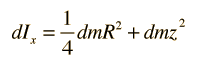Now expressing the mass element dm in terms of z, we can integrate over the length of the cylinder.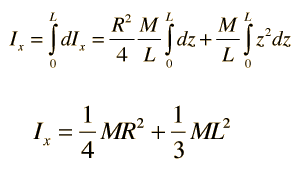This form can be seen to be plausible it you note that it is the sum of the expressions for a thin disk about a diameter plus the expression for a thin rod about its end. If you take the limiting case of R=0 you get the thin rod expression, and if you take the case where L=0 you get the thin disk expression.

The last steps make use of the polynomial forms of integrals.

### Thin disc moments of inertia

Index

Moment of inertia concepts

 HyperPhysics***** Mechanics R Nave
Go Back